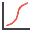Join one of our upcoming courses taking place around the world! Explore now

# Cumulative Plot

A cumulative plot (also called S-curve) shows the probability that a variable will have a value less than or equal to a specified value.

To create a Cumulative plot:

1. Select Cumulative Plotfrom the toolbar or the Statistics menu.
2. From the dialog that appears, select the Variable to Plot and Number of Bins (see below for details).
3. You may also choose the following plotting option, using the check box provided. See the Plotting Options topic for details.
• Attach Markers
4. Click OK to create the plot.

## Variable to Plot

The user can create a cumulative plot for any calculated (output) variable, or for any input variable that has a statistical distribution. In the Variable to Plot list, the output variables are always listed first (e.g. Factor of Safety, Tunnel Convergence), followed by the input random variables (e.g. In-Situ Stress, Young's Modulus, etc.)

## Number of Bins

Number of Bins determines the number of intervals between the minimum and maximum values of the variable. The maximum allowed number is 500. Note that if the interval size becomes too small for the program to display, number of bins is automatically reduced to one.